In this article I'm going to try to provide some intuition around how multivariate Gaussian distributions and Gaussian process models can be useful for modeling smooth functions.

A random variable $X$ in $d$ dimensions is multivariate normally distributed e.g. $X \sim \cal N_d(\mu, \Sigma)$ if and only if $\Sigma_i \alpha_i X_i \sim \cal N(\mu', \sigma')$ for any choice of $\{ \alpha_i \}$ and $i$ taken across any subset of $\{1, ..., d\}$ for some $\mu'$ and $\sigma'$. In other words, the sum or linear combination of any subset of a multivariate normal distribution's components is a normal distribution.

### Understanding the Role of the Covariance Matrix

It’s useful to consider the extremal situations first.

Here’s a draw from a multivariate normal distribution where the mean is 0 and the covariance matrix is all 1s: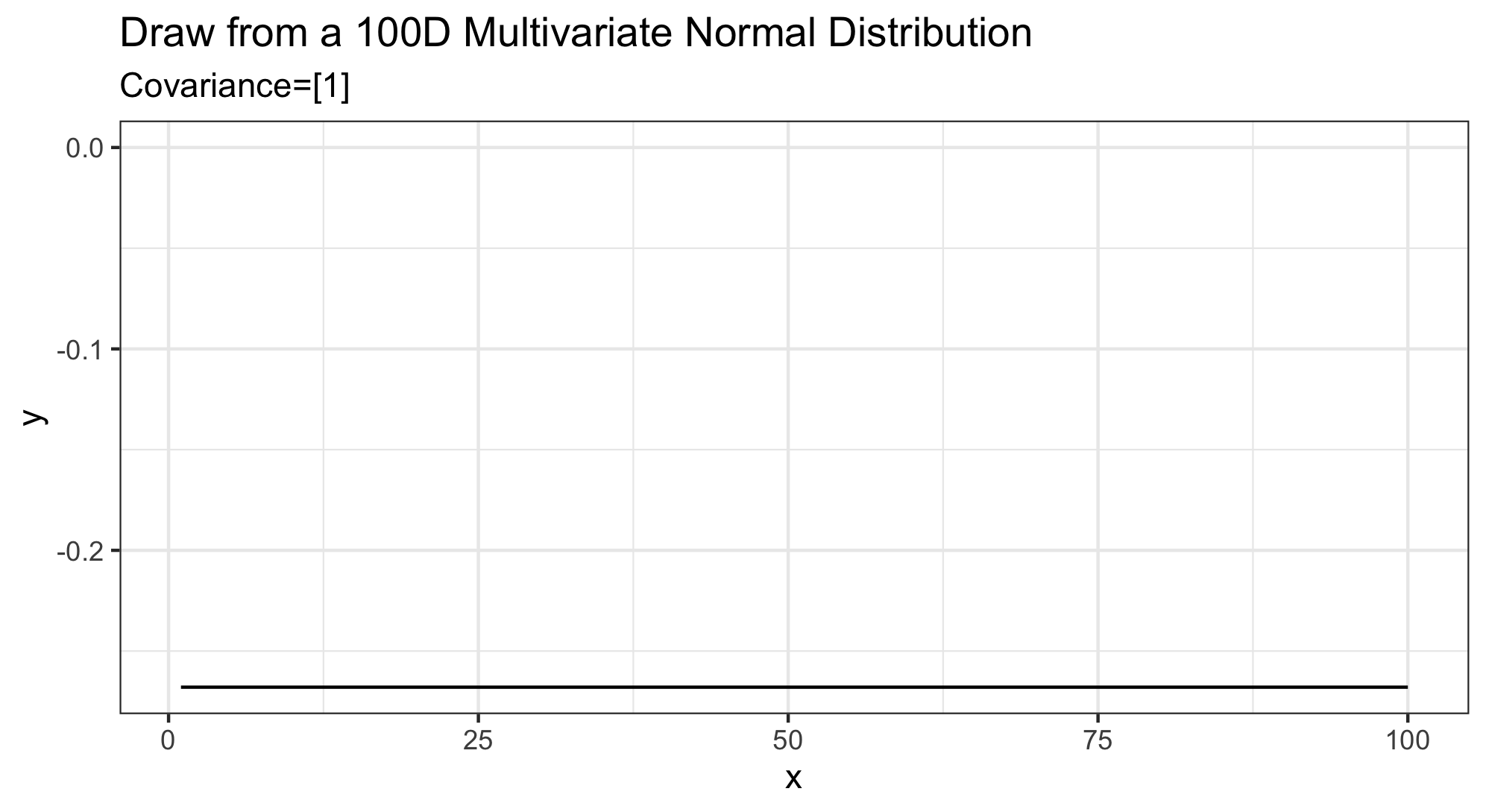The covariance matrix being full of ones forces all elements of the vector to be exactly the same.

The other extreme is when the covariance matrix is 1 on the diagonal entries and 0 everywhere else. Here’s a draw from that distribution, again with mean 0: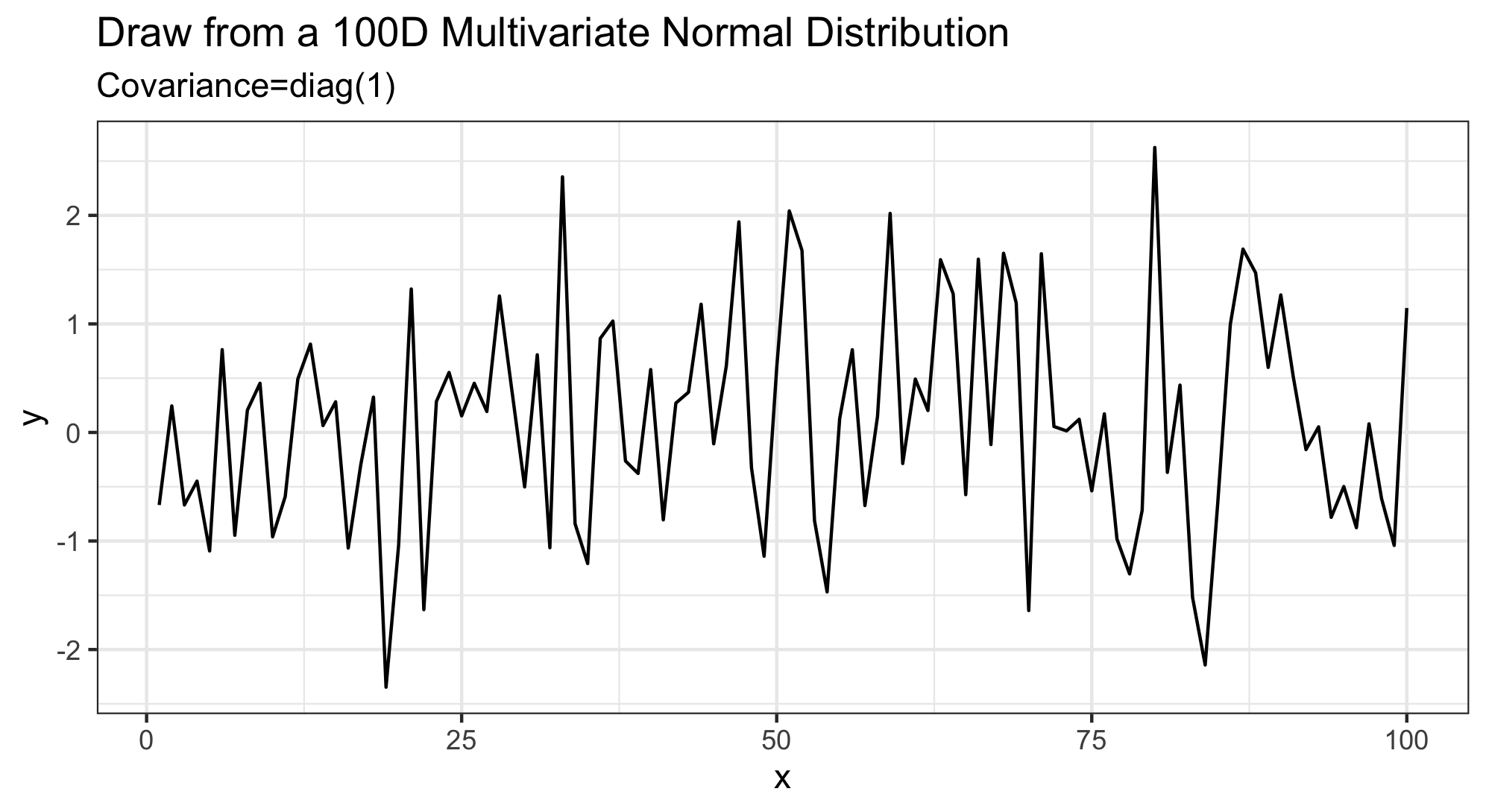This results in a very noisy vector which is still roughly centered at 0.

Another easy covariance matrix to specify is where the off-diagonal elements are all some fixed number, say .999: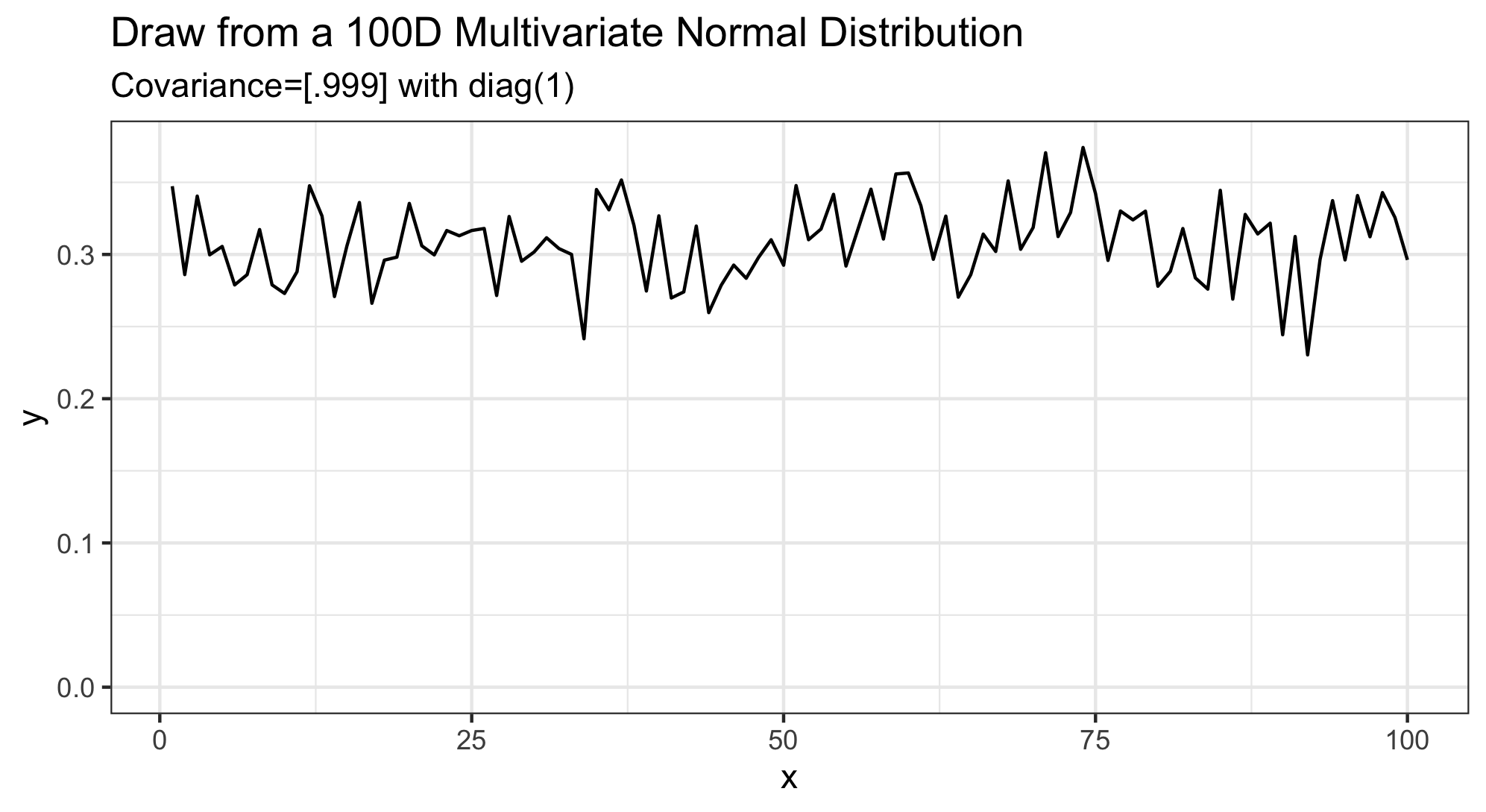This results in a noisy vector, but where all the entries are correlated with each other, resulting in reduced deviation within the components of the draw.

### Using Gaussian Kernels

Now that we understand how the multivariate normal distribution acts given some examples of covariance matrices, we can think about more practical situations such as when the covariance matrix is defined based off a kernel function like the Gaussian kernel.

The Gaussian kernel is defined as $K(x_1, x_2) = \text{exp}(-\frac{1}{\rho}(x_1-x_2)^2)$ for some choice of smoothing parameter $\rho$.

Given $X = [0, 0.1, 0.2, ..., 10]$ we can define our covariance matrix as $[K(X_i,X_j)]_{\{i,j\}}$. Essentially this results in a covariance matrix where neighboring components (and nearby components) are expected to be highly correlated while more distant components are expected to have less correlation.

First, here’s an example with $\rho=1$: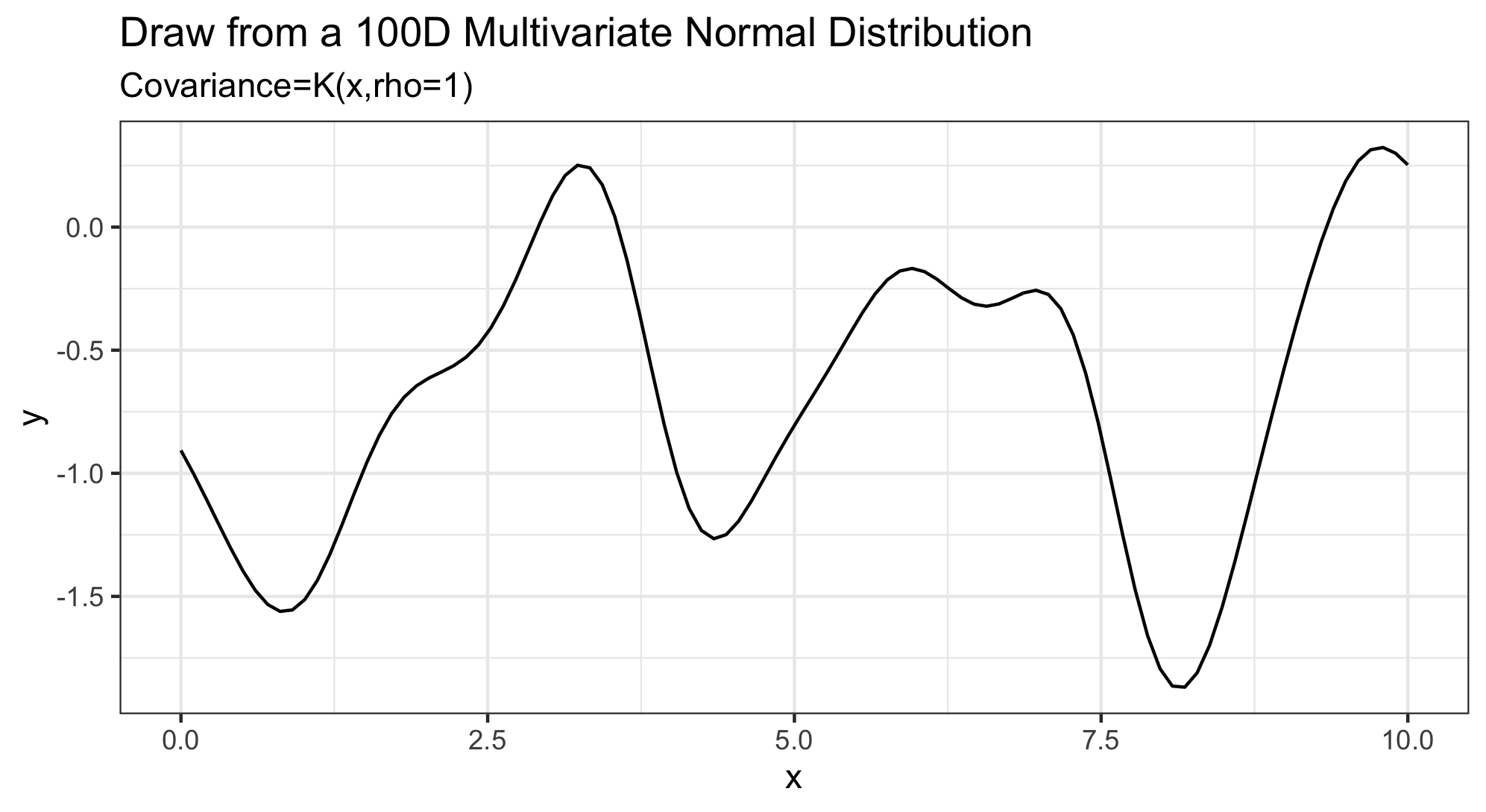If we reduce $\rho$ to .1 we get more wiggles: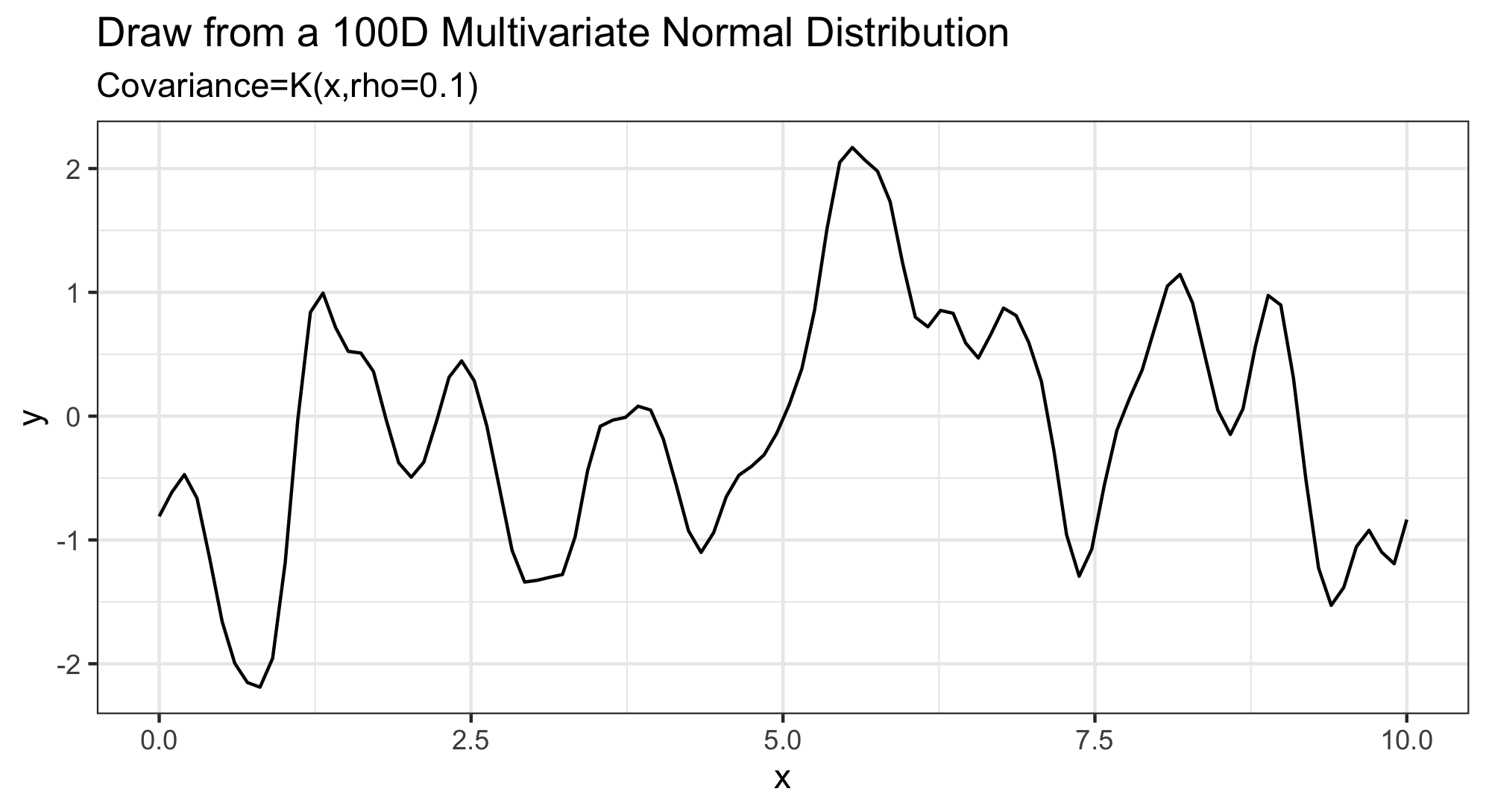### Function Approximation using MVNs and the Gaussian Kernel

It’s useful to note that throughout the Bayesian Statistics literature one can find frequent references to “Gaussian Processes” which are defined as having a prior distribution given by a multivariate normal distribution. Gaussian Process models have made quite the splash in areas of machine learning and statistics as a flexible means of modeling smooth surfaces. Sometimes Gaussian Process Models also go by the name of “kriging” which commonly comes up in geospatial statistics.

To create an example scenario in which we can apply some Gaussian Process modeling, let’s define a wiggly function from which we will take some sample observations. Then we will fit the data using a Gaussian Process model and see how the model compares to the true function. We won’t take model assessment too seriously here, but we will qualitatively compare the Gaussian Process model’s performance to other common techniques like cubic splines and locally weighted smoothing (loess).

h_func <- function(x) { cos(x^2) + x }
n <- 25
X <- runif(n,0,4)
h_vals <- h_func(X)
h_mean <- mean(h_vals)
h_vals <- h_vals - h_mean

Note that we’re centering the data so that it’s compatible with our later assumption that $\mu = 0$ for our Gaussian Process model.